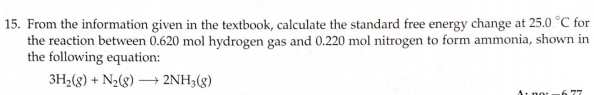# 15. From the information given in the textbook, calculate the standard free energy change at 25.0 degrees C for the reaction between 0.620 mol hydrogen gas and 0.220 mol nitrogen to form ammonia, shown in the following equation: 3H2(g) + N2(g) → 2NH3(g)